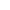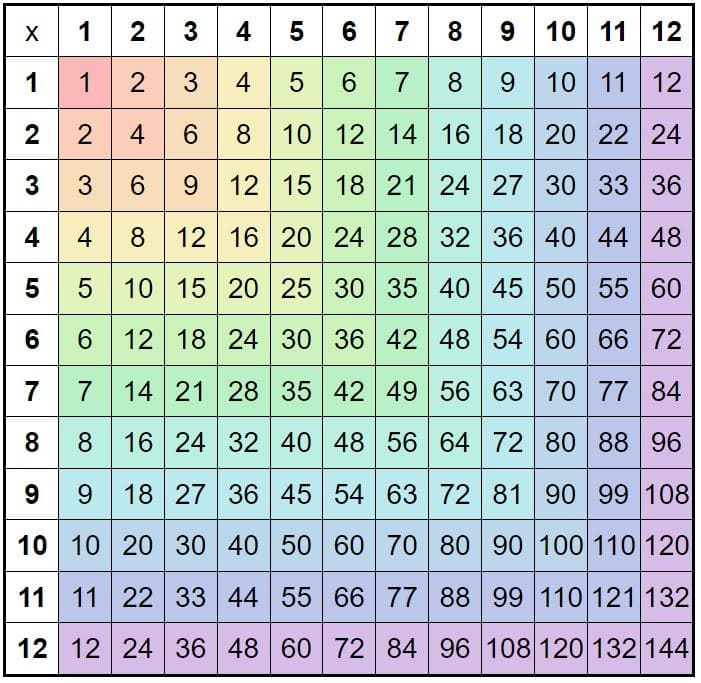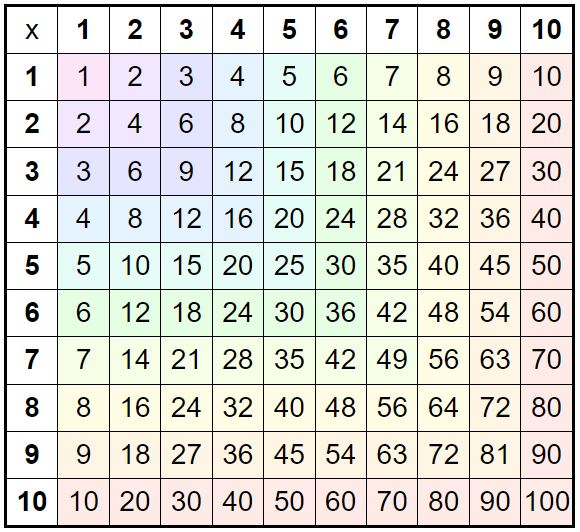﻿ Printable Multiplication Chart + Interactive Games

Page No. 1063

# Multiplication chart

Online multiplication chart to 100. 12 times table chart. Download free printable multiplication chart 1 12 printable. Times table grid. multiply chart multiplication table. Multiplication 1-100 chart. multiplication grid printable. Free printable multiplication chart 1 20 PDF. Table chart 1 to 20. Play interactive multiplication chart games. 1 to 100 tables chart PDF. Blank multiplication chart free printable. Free printable multiplication chart with missing numbers PDF. 100x100 multiplication chart printable 1 12. Math table chart printable. Free multiplication chart printable. free online multiplication square interactive.

New gameFill in the missing number in the multiplication chart.

## Interactive multiplication chart 1-100Fill in the missing number in this interactive multiplication chart game, then press "Check it" to find out if the multiplication grid is filled in correctly. The correct answer will be green, wrong outcome will be red. Press the button "New game" and the new times table chart will be generated.

## Free printable multiplication chart 1-12

The printable multiplication grid can be used to quickly find the products of any two numbers from 1 to the number of the chart (10, 12 or 20) without having to do the actual calculation.

Download our free printable multiplication chart in PDF below or browse all multiplication worksheets here.

## Multiplication grid method

Interactive multiplication chart is a table with 100 squares arranged in a 10x10 grid.

To use a printable multiplication chart, find the row and column that correspond to the two numbers (factors) you want to multiply. The product of those two numbers will be found at the intersection of that row and column.

For example, if you wanted to know what 3 x 7 is, you would look for the row labeled "3" and the column labeled "7", and the intersection of those would be 35. Kids can easily memorize the multiplication table this way.

### What is a multiplication chart?

A multiplication chart, also known as a times table or multiplication table, is a structured grid used to display the results of multiplying two numbers within a specific range.

A multiplication grid is a useful tool for quickly finding the product of two numbers (factors) without performing the actual multiplication operation.

The factors are the numbers in the top row and leftmost column, the cells within the multiplication chart contain the products of the corresponding multipliers.

## How can a multiplication table chart be useful?

Here are a few examples how can you use the multiplication chart:

• Quickly finding the product of two numbers: 🌸 If you need to multiply two numbers and don't have a calculator, a multiplication chart can be a lifesaver. Just find the row with one number and the column with the other, and you'll have your answer.
• Reviewing multiplication facts: 🌸 Our interactive multiplication chart game can be a great way to review the facts you've learned. Just cover up one column or row and try to fill in the missing products.
• Teaching multiplication: 🌸 If you're a teacher, you can use a multiplication grid to introduce the concept of multiplication to your students. You can also use it to help students practice and memorize multiplication facts.

In summary, together with online multiplication chart 1-100 is the printable multiplication grid a handy reference tool that can be used for quickly finding products, reviewing multiplication facts, and teaching single digit multiplication.

## Online multiplication chart to 100

An interactive multiplication chart with missing numbers is a digital version of the traditional printable multiplication table chart, but it serves for reviewing multiplication facts. Students must fill in the missing products by performing the multiplication themselves.

Interactive multiplication charts can be a useful tool, as they can provide an engaging way to learn and practice multiplication.

Don´t forget to practice multiplication also by using printable multiplication games, multiplication cards, and other interactive activities such as multiplication puzzles.## Times table chart for students

There are really many benefits of using multiplication charts in math lessons.

If you're a student, parent, or teacher, you know how important it is to have a reliable printable multiplication chart at hand.

Whether you're working on math homework, preparing for a test, or teaching a lesson, a multiplication grid can be a valuable tool.

A multiplication chart is an effective tool to help learn multiplication, particularly for younger students or those who are just starting to learn the multiplication tables. Students can see the patterns and relationships between the numbers in a times table chart and learn the multiplication facts through repetition and memorization.

## 100 multiplication chart

For children who need to understand the principle of multiplication, I have prepared multiplication arrays games.

A multiplication chart and a multiplication array are both tools that can be used to help visualize and learn multiplication tables. However, they are slightly different in their presentation and use:

Multiplication arrays are rectangular grids of squares that represents a multiplication problem by using rows and columns of dots or symbols. Each row and column represents one of the factors in the multiplication problem, and the number of dots in each row and column represents the value of that factor. The product of the two factors is represented by counting the total number of dots in the array.

For example, a multiplication array for 4x5 would be a rectangle divided into four rows and five columns, with a total of 20 dots arranged in a grid. Each row would have four dots, and each column would have five dots.

### Free multiplication chart

In summary, while both a multiplication chart and a multiplication array are useful tools for learning and practicing multiplication, the times table chart presents the products in a table format while the array represents the multiplication problem using rows and columns of dots or symbols.